New Foundations Explorer < Previous   Next > Nearby theorems Mirrors  >  Home  >  NFE Home  >  Th. List  >  ax11o Unicode version

Theorem ax11o 1994
 Description: Derivation of set.mm's original ax-11o 2141 from ax-10 2140 and the shorter ax-11 1746 that has replaced it. An open problem is whether this theorem can be proved without relying on ax-16 2144 or ax-17 1616 (given all of the original and new versions of sp 1747 through ax-15 2143). Another open problem is whether this theorem can be proved without relying on ax12o 1934. Theorem ax11 2155 shows the reverse derivation of ax-11 1746 from ax-11o 2141. Normally, ax11o 1994 should be used rather than ax-11o 2141, except by theorems specifically studying the latter's properties. (Contributed by NM, 3-Feb-2007.)
Assertion
Ref Expression
ax11o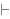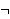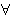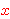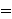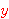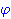Proof of Theorem ax11o
Dummy variable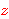is distinct from all other variables.
StepHypRef Expression
1 ax-11 1746 . 221ax11a2 1993 1Colors of variables: wff setvar class Syntax hints:wn 3wi 4wal 1540 This theorem was proved from axioms:  ax-1 5  ax-2 6  ax-3 7  ax-mp 8  ax-gen 1546  ax-5 1557  ax-17 1616  ax-9 1654  ax-8 1675  ax-6 1729  ax-7 1734  ax-11 1746  ax-12 1925 This theorem depends on definitions:  df-bi 177  df-an 360  df-tru 1319  df-ex 1542  df-nf 1545 This theorem is referenced by:  ax11b  1995  equs5  1996  ax11v  2096
 Copyright terms: Public domain W3C validator